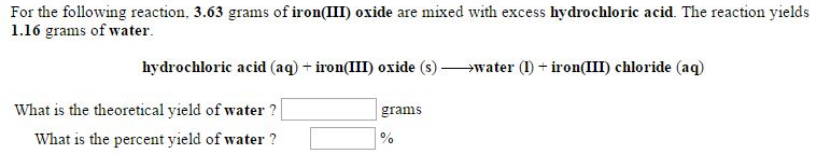# Problem: For the following reaction, 3.63 grams of iron(III) oxide are mixed with excess hydrochloric acid. The reaction yields 1.16 grams of water. hydrochloric acid (aq) + iron(III) oxide (s) → water (I) + iron(III) chloride (aq) What is the theoretical yield of water? What is the percent yield of water?

###### FREE Expert Solution
100% (413 ratings)###### Problem Details

For the following reaction, 3.63 grams of iron(III) oxide are mixed with excess hydrochloric acid. The reaction yields 1.16 grams of water.

hydrochloric acid (aq) + iron(III) oxide (s) → water (I) + iron(III) chloride (aq)

What is the theoretical yield of water?

What is the percent yield of water?